# Bihar Board 12th Economics Model Question Paper 1 in English Medium

Bihar Board 12th Economics Model Papers

## Bihar Board 12th Economics Model Question Paper 1 in English Medium

Time : 3 Hour 15 Min
Full Marks : 100

Instructions

1. Candidates are required to give their answers in their own words as far as practicable.
2. Figures in the right hand margin indicate full marks.
3. 15 minutes of extra time has been allotted for the candidate to read the questions carefully.
4. This question paper has two sections : Section-A and Section-B.
5. In Section-A, there are 50 objective type questions which are compulsory, each carrying 1 mark. Darken the circle with black/blue ball pen against the correct option on OMR Sheet provided to you Do. not use Whitener/Liquid/Blade/Nail on OMR Sheet, otherwise the result will be treated as invalid.
6. In Section-B, there are Non-objective type questions. There are 25 Short answer type questions, out of which any 15 questions are to be answered. Each question carries 2 marks. Apart froms this, there are 08 Long answer type questions, out-of which any 04 of them are to be answered. Each questions carries 5 marks.
7. Use of any electronic device is prohibited.

Objective Type Questions

Questions no. 1 to 50 have four options provided out of which only one is correct. You have to mark, your selected option, on the OMR-Sheet. Each question carries 1 (one) marks.

Question 1.
Production Possibility curve is :
(a) Concave to the axis
(b) Convex to the axis
(c) Parallel to the axis
(d) Vertical to the axis
(a) Concave to the axis

Question 2.
Which one is the limitation of Macro Economics ?
(a) Collective Economic
(c) Both A and B
(d) None of these
(c) Both A and BQuestion 3.
How we calculate marginal utility ?
(a) $$\frac { ΔMU }{ ΔQ }$$
(b) $$\frac { ΔTU }{ ΔQ }$$
(c) $$\frac { ΔQ }{ ΔTU }$$
(d) $$\frac { ΔQ }{ ΔMU }$$
(b) $$\frac { ΔTU }{ ΔQ }$$

Question 4.
Who propounded the ordial utility theory ?
(a) Marshall
(b) Pigou
(c) Recardo
(d) Hicks & Allen
(d) Hicks & Allen

Question 5.
The quantity of goods which the seller is ready to sell in a market at fixed price and time is called.
(a) Supply
(b) Demand
(c) Elasticity of supply
(d) Elasticity of Demand
(a) Supply

Question 6.
Which is a reason of change in demand ?
(a) Change of consumer’s income
(b) Change in price of related goods
(c) Population Increase
(d) All of these
(d) All of these

Question 7.
For Giffin goods, price elasticity of demand is :
(a) Negative
(b) Positive
(c) Zero
(d) None of these
(b) Positive

Question 8.
Production function is expressed as :
(a) QX = PX
(b) QX = DX
(c) QX = f (A,B,C,D)
(d) None of these
(c) QX = f (A,B,C,D)

Question 9.
Which of the following is not fixed cost ?
(a) Cost of raw materials
(b) Rent of the factory
(c) Interest
(a) Cost of raw materials

Question 10.
Which of the following is true ?
(a) TVC = TC – TFC
(b) TC = TVC – TFC
(c) TFC = TVC – TC
(d) TC = TVC × TFC
(a) TVC = TC – TFC

Question 11.
In which market MR may become zero or negative ?
(a) Monopoly
(b) Monopolistic competition
(c) Both A and B
(d) Perfect competition
(c) Both A and B

Question 12.
In perfect competition :
(a) AR = MR
(b) AR > MR
(c) AR < MR
(d) AR + AC = MR
(a) AR = MRQuestion 13.
The basic condition of a firm’s equillibrium is :
(a) MR = AR
(b) MC > MR
(c) MR < TR
(d) AC = AR
(b) MC > MR

Question 14.
If the price of goods rises by 20% and supply increases by 40%, then the supply of such goods will be :
(a) Elastic
(b) Inelastic
(c) Highly Elastic
(d) Perfectly Elastic
(d) Perfectly Elastic

Question 15.
Which one of the following is true for monopoly.
(a) Firm is price-maker
(b) Demand curve slops downward
(c) Price discrimination Possibility arises
(d) All of the above
(d) All of the above

Question 16.
Who gave the concept of ‘Time Element’ in price determination process ?
(a) Ricardo
(b) Walras
(c) Marshall
(d) J.K. Mehta
(c) Marshall

Question 17.
Which is the component of factor price determination ?
(a) Rent
(b) Wages
(c) Interest
(d) All of the above
(d) All of the above

Question 18.
The elasticity of a straight line supply curve originating from the centre of origin is
(a) Less than unit
(b) Greater than unit
(c) Equal to unit
(d) Equal to Zero
(c) Equal to unit

Question 19.
The alternative name of opportunity cost is :
(a) Equilibrium Price
(b) Economic Cost
(c) Marginal Cost
(d) Average Cost
(b) Economic Cost

Question 20.
What happens when production is shut down ?
(a) Variable costs become zero
(b) Fixed costs increases
(c) Variable costs decline
(d) Fixed costs become zero
(a) Variable costs become zeroQuestion 21.
A curve which rises first and starts declining after that is called :
(a) APP
(b) MPP
(c) TPP
(d) All these
(d) All these

Question 22.
An active factor of production is :
(a) Capital
(b) Labour
(c) Land
(d) None of these
(c) Land

Question 23.
Which of the following is the main reason of operating the Law of Dimishing Returns ?
(a) Scarcity of factors
(b) Imperfect Substitution between Factors
(c) Both A and B
(d) None of these
(c) Both A and B

Question 24.
Average variable costs is :
(a) TVC × Q
(b) TVC + Q
(c) TVC – Q
(d) TVC ÷ Q
(d) TVC ÷ Q

Question 25.
“Supply creates its own demand”. Who propounded this law ?
(a) J.B. Say
(b) J.S. Mill
(c) Keynes
(d) Ricardo
(a) J.B. Say

Question 26.
With increase in investment, MEC :
(a) Rises
(b) Falls
(c) Remains Constant
(d) None of these
(b) Falls

Question 27.
Increase in aggregate demand of equilibrium level of income and employment causes increase in :
(a) Employment
(b) Production
(c) Income
(d) All of the above
(d) All of the above

Question 28.
Which one is correct ?
(a) A.K = $$\frac { 1 }{ MPc }$$
(b) B.K = $$\frac { 1 }{ MPc }$$
(c) C.K = $$\frac { 1 }{ 1-MPc }$$
(d) D.K = $$\frac { 1 }{ 1+MPs }$$
(b) B.K = $$\frac { 1 }{ MPc }$$

Question 30.
Which of the following is included in secondary sectors ?
(a) Insurance
(b) banking
(d) Investment
(d) Investment

Question 31.
Net national income at Factor cost is called :
(a) National Income
(b) Gross Investment
(c) Domestic Income
(d) None of these
(a) National IncomeQuestion 32.
The market value of all final goods and services produced in an economy over a year is called :
(a) Gross National Product
(b) National Income
(c) Gross Domestic Product
(d) Net National Product
(c) Gross Domestic Product

Question 33.
Which service is included in Tertiary Sector ?
(a) Mining
(b) Communication
(c) Construction
(d) Animal Husbandry
(b) Communication

Question 34.
GNPMP = ?
(a) GNPMP – Depreciation
(b) GNPMP + Net Factor Income from abroad
(c) GNP + Subsidy
(d) None of the above
(b) GNPMP + Net Factor Income from abroad

Question 35.
Which of the following is correct ?
(a) Disposable Income = Personal Income – Direct Taxes
(b) Disposable Income = Private Income – Direct Taxes
(c) Disposal Income = Personal Income – Indirect Taxes.
(d) Disposal Income = Private Income – Indirect Tases
(a) Disposable Income = Personal Income – Direct Taxes

Question 36.
Which Objectives government attempts to obtain by Budget ?
(a) To Promote Economic Development
(b) Balanced Regional Development
(c) Redistribution of Income and Wealth
(d) All the above
(d) All the above

Question 37.
Which of the following Budget is suitable for Developing Economy ?
(a) balanced Budget
(b) Surplus Budget
(c) Deficit Budget
(d) None of these
(c) Deficit Budget

Question 38.
Foreign exchange is determined by :
(a) Government
(b) Bargaining
(c) World Bank
(d) Demand and supply forces
(d) Demand and supply forces

Question 39.
“Budget is a preliminary approval Plan of Public revenue and expenditure”. Who said?
(a) Rane stourn
(b) Findlay Shirras
(c) Taylor
(d) Dalton
(d) Dalton

Question 40.
“Money is what mobey does” who said it ?
(a) Hartley Withers
(b) Hawtrey
(c) Prof Thomas
(d) Keynes
(a) Hartley Withers

Question 41.
The function of money is
(a) Medium of exchange
(b) Measure of value
(c) Store of value
(d) All of the above
(d) All of the above

Question 42.
What is Central Bank of India ?
(a) Central Bank
(b) Commercial Bank
(c) Private Bank
(d) Co-operative Bank
(b) Commercial Bank

Question 43.
Who regulates money supply ?
(a) Govt, of India
(b) Planning Commission
(c) Reserve Bank
(d) Commmercial Bank
(b) Planning CommissionQuestion 44.
Who is the Banker’s of the Bank ?
(a) Reserve Bank of India
(b) State Bank of India
(c) Unit Trust of India
(d) None of the above
(a) Reserve Bank of India

Question 45.
In which year Banking Ombudsman Scheme was announced ?
(a) 1990
(b) 1955
(c) 1977
(d) 1988
(b) 1955

Question 46.
Which type of currency is issued by the central Bank ?
(a) Currency
(b) Credit Money
(c) Coins
(d) All the above
(a) Currency

Question 47.
Which items are included in Balance of Payments?
(a) Visible items
(b) Invisible items
(c) Capital Transfer
(d) All the above
(d) All the above

Question 48.
(a) Capital Transaction
(b) Import Export of goods
(c) Total Debit and Credit
(d) All of the above
(b) Import Export of goods

Question 49.
The meaningt of Spot Market relates to foreign exchange is ?
(a) Current Transactions
(b) Transactions of mid-period
(c) Transactions of Long Term
(d) None of these
(a) Current Transactions

Question 50.
The Hybrid systems of exchange rate related to
(a) Wider Bands
(b) Crawing peg
(c) Managed Floating
(d) All of them
(d) All of them

Non-Objective Type Questions

Question no. 1 to 25 are Short answer type questions. Answer any 15 them in 50 words. Each question carries 2 marks. (15 x 2 = 30)

Question 1.
What do you understand by Micro Economics ?
Small units of an economy are included in micro economics e.g. one consumer, one producer, one firm, one industry etc. In other words, individual units are studied in micro economics.

According to K.E. Boulding, “Micro economics may be defind as that branch of analysis, which studies the economics behaviour of the individual unit, may be a person, a particular house-hold or a particular firm. It is a study of one particular unit rather than all the units combined together,”

Question 2.
Write two main components of Macro Economics ?
The term Macro in English shas its origin in the Greek term Macros which means large. In the context of macro economics “large” means economy as a whole. Thus, macro economic is defined as that branch of economics which studies economic activies at the level of an economy as a whole.

According to M.S. Spencer, “Macro-economics is concerned with the economy as a whole or large segments of it. In Macroeconomics, attention is focused on such problems as the level of unemployment, the rate of inflation, the nation’s total output and other maters, of economy-wide significance.”

Question 3.
Distinguish between utility and total utility. Ans. Demand schedulers that schedule which, other things, remaining constant, expresses the relation between different quantities of the commodity demanded at different prices. The table relating to price and quantity demanded is called the demand schedule.
Demand schedule is of two types :

• Individual demand schedule
• Market demand schedule.
1. A more and more units of a commodity are consumed the marginal utility derived from each successive unit goes on diminishing. But the total utility increases up a limit.
2. Marginal utility of the first four units, being positive the total utility goes on increasing thus, as long as the marginal utility of the commodity remains positive, total utility goes on increasing.
3. Marginal utility of the sixth unit is negative (-2). As a result of it, total utility of six units of the commodity falls from 20 to to 1 units.
Thus,

1. When MU diminishes, TU tends to increase at a diminishing rate
2. When MU is zero, Tu is maximum
3. When MU is negative, TU starts diminishing.Question 4.
What is indifference curve ?
Ans. Indifference curve explains the consumer’s behaviour related with the combination of two goods and this consumer’s behaviour is explained with the help of “Indifference schedule or Indifference set.” various combinations of two goods giving equal satisfactions to the consumer become the component of ‘Indifference schedule’, When indifference schedule is represented on a graph paper, we get indifference curve.

In the words of watson “An indifference schedule is the lists of combination of two commodities, the list being so arrange that a consumer is indifferent to the combinations preferring more of any other”.

Question 5.
What are the exceptions of law of Demand ?
There are following to the Law of Demand.

1. Expected rise in future price
2. Prestigious Goods
3. Ignorance of consumer

Question 6.
Explain any two factors that affect price elasticity.
Factors affecting elasticity of demand are discussed below.

1. Substitutes : When substitute of any goods is available, then deamnd elasticity of such goods is highly elastic because when price of these goods increases, subsutities are used in place of it. In the same way on decrease in price of these goods, use of substitutes decrease. Tea, coffee, gas, sugar etc. are examples of subsitutes.
2. Alternative uses : When goods could be used in only one way, its demand will be elastsic and when it would be used in many ways, its demand will be elastic eg. coal has so many used-it can be used industries houses ralways etc. Demand of coal for railways in elastic but for house purpose, its cheeper alternative goods like wood cooking gas etc. could be used in place of it.

Question 7.
What do you understand by Production Function ?
A Production expresses the technical relationship between input and output of a firm. It tells us about the maximum quantity of output that can be produced with any given quantities of inputs. If there are two factor inputs, labour (L) and capital (K), then production function can be written as :
Q = f (L, K) where
Q = Quantity of output
L = Units of labour
K = Units of capital
It may be pointed here that both the inputs are necessary for the production. If any of the inputs is zero, there will be no production with both inputs and output will also be zero, As we increase the amount of any one input, output increases.

Question 8.
What is difference between Explicit Cost and Implicit Cost ?
Explicit cost: Explicit costs refer to the actual money outlay of the firm to buy or wire the productive resources, it needs in the process of proudction.
Implicit Costs : Implicit costs are payments which are not directly or actually paid out by the firm as no contractual disbursement is fixed for them. It includes the prices of such services and factors which are used in production process but are not directly paid.

Question 9.
Why does a firm attain maximum Profit When MR = MC ?
MC stands for marginal cost incurred by a firm when its production raises by one unit. If the marginal cost is smaller than the marginal revenue (MR), then it is profitable for the firm to produce on extra Unit of output.

Question 10.
What are the different types of supply elastiicity ?
Following are the types of elasticity supply.

1. Perfectly Elastic Supply (eV = ∞)
2. Greater than unitary Elastic supply (es> 1)
3. Unitary Elastic Supply (es = 1)
4. Inelastic Supply (es < 1)
5. Perfectly Inelastic supply (es = 0)Question 11.
Write three main features of monopoly market.
Features of monopoly are as under-

1. One seller and large number of buyers : Under monopoly, there should be a single producer of a commodity. He may be alone or there may be a group of partner or a joint stock company. Thus, there is only one firm under monopoly. But the buyers of the product are in large number.
2. Restrictions on the entry of the new firms : Under monopoly, there are some estrictions on the entry of new firms into monopoly industry.
3. No close substitutes : A monopoly firm produces, a community that has close substitutes.
4. Full control over price : A monopolist has full control over its price. A monopolist thus, is a price maker. He can fix whatever price he wishes to fix for his product.
5. Possibility of price discrimination : A
monopolist charges different prices from different consumers. It is called price descrimination. It refers to the practice by a seller of charging different prices from different buyers for the same good.

Question 12.
Explain the Circular Flow of Income.
Production gives birth to income, income to consumption, consumption to ependiture and again expenditure to income and production. Thus, circular flow of income earning economic activities takes place in the economy.
According to hipster, “The circular flow of income is the flow of payment and receipt between domestic firms and domestic households.”

Hence, the circular flow of income refers to flow of money income or the flow of goods an services across different sectors of the economy in a circular form.

Question 13.
What are the uses of National Income Accounting ?
The information collected through nation income accounting can be used for a variety of purposes, such as assessing the current standard of living or the distribution of income within a population. Additionally, national income accounting provides a method for comparing activities within different sectors in an economy, as well an changes within those sectors over time. A thorough anlysis can assist in determining overall economic stability within a nation.

Question 14.
What is the relationship between Gross Domestic Product and Gross national Product.
Distinction between GDP and GNP :Question 16.
What is the difference between Standard money and Token Money ?
The Indian rupee is a mixture of the standard money and the token money. Like standard money. It is unlimited legal tender, and like the token money, its face value is greater than its intrinsic value.Question 17.
State the qualiity of good money.
Following are the qualities of good money :

1. Utility : The metal with which coin is made, must bear the feature of utility. The metal must easily be accepted. Gold and silver are such metals which possess utility.
2. Port ability : The metal with which coin is made, can easily be tranferred from one place to antother. Gold and silver coins bear this feature also.
3. Durable : Money is saved by people and hence coin should be made of such metal which is durable from saving purpose.
4. Divisibility : Money metal should be divisible without my loss in its value. Gold and silver are such metals having the feature of divisibility.
5. Homogeneity : All units, of money should be homogeneous.
6. Economy : Minting cost’of coin should be minimum and the depreciation in the coin should be least.
7. Stability of Value : Money metal should be stable in value i.e. price fluctuation in the metal of coin should be minimum.
8. Liquidity : Money metal should be liquid in nature, metal should easily be converted into coins can again be converted into meta on easily.
9. Cognisibility : Metal should easily be indentified. Take coins on easily be traced out if metal of money is cognisible. Gold and silver are such metals having full cognisibility.

Question 18.
What is difference between cash credit and overdraft ?
At the simplest level, overdraft and cash credit are just forms of borrowing. The institution allows you to withdrew funds that you do not have, usually in small amounts. The primary differences between these forms of borrowing is how they are secured and whether the money is lent out of a separate account.

Question 19.
State the types of Government Budget.
Following are the types of Budget.

1. Balanced Budget.
2. Deficit Budget.
3. Surplus Budget.

Question 20.
Mention any two participants of the Foreign Exchange Market.
Following are the two participants of foreign exchange market.

1. Central Banks.
2. Foreign Exchange Brokers.

Question 21.
What is unfavorable Balance of Payment.
Unequal flow of money under an unfavorable balance of payments will reduce the country’s money supply and increase the exchange rate to currencies in other countries, causing issues with inflation, unemployment and production. Also called balance of payments deficit.

Question 22.
Explain the relationship between investment Multiplier and Marginal Propensity to consume.
The Multiplier Effect. The marginal propensity to consume (MPC) is equal to AC/AY, where AC is change in consumption, and AY is change in income. If consumption increases by eighty cents for each additional dollar of income, them MPC is equal to 0.8/1 = 0.8.

Question 23.
When does demand become Inelastic ?
Demand becomes inelastic when people by about the same amount whether the price drops or rises. That happens with things people must have, like gasoline. Drivers must purchase the same amount even when the price increases. Like wise, they don’t buy much more even if the price drops.

Question 24.
Explain two features of oligopoly ?
Important features of oligopalistic market are :

1. Few sellers
2. Interdependence among sellers regarding policies about price and output determination.

Question 25.
What is meant by Revenue Deficit ?
Revenue deficit is the excess of revenue expenditure over revenue receipts. Revenue receipts include both tax revenue and non-tax revenue. Similarly revenue expenditures also include both plan and non-plan expenditures on revenue account. Revenue dificit does not include items of capital receipts and capital expenditure.
[Revenue Deficit] = [Revenue Expenditur] – [Revenue Receipts]

Question no. 26 to 33 are Long answer type questions. Answer any 4 of them in 100 words. Each question carries 5 marks. (5×4 = 20)

Question 26.
Explain the law of Demand. What are its assumptions ?
Law of demand-Low of demand explains quantitative relation behueen price of goods and quantity demanded. Every consumer has a psychology to buy less amount or quantity of anything of high price and more quantity of low price, ceteris Paribus (others being equal), there is inrerse relationship between price of a goods and when price is low, demand is high.

Thus, P ∞$$\frac { 1 }{ Q }$$ where, P = Price of commodity, Q = Quantity demanded
Assumptions of the law of demand-Law of demand is based on few assumptions. These assumptions are-

1. Consumer’s income should remain contant.
2. Consumer’s taste, nature, etc. should remain constant
3. Price of related goods should remain constant
4. Consumer remains unknown with new substitutes
5. There is no possibility of price change in future.Question 27.
Explain the factors determining elasticity of demand.
Factors affecting Elasticity of Demand :

1. Substitutes : When substitutes of any goods is available, then demand elasticity of such goods is highly elastic, because when price of these goods increases, substitutes are used in pace of it. In the same way, on decrease in price of these goods, use of substitutes decreases. Tea, coffee, sugar, etc. are examples of substitutes.
2. Alternative Uses : When goods could be used in only one way, its demand will be inelastic and when it would be used in many ways, its demand will be elastic. For example, coal has many uses-it can be used in railways, houses, industries, etc. Demand of coal for railway is inelastic but for house purpose, its demand is elastic as many cheaper alternative goods like wood, cooking gas etc. could be used in place of it.
3. Postponement of Consumption : If consumption of any goods can be postponed, its demand becomes elastic, e.g., a person has to woolen clothes. If due to some reason, price of woolens clothes increases, then consumer postpones the idea of buying another sweater in current year. In such a case, demand of woolen clothes for current year will be highly elastic.
4. Expenditure Amount: Goods on which large part of income is spent, are highly demand elastic and those goods on which small part of income is spent, their demand is generally inelastic. That is why, demand of television, radio, cycle, scooter, etc. is more elastic as compared to salt, wheat etc.
5. Income Level : Elasticity of demand for rich people is generally inelastic because increase in price level does not affect them severly while elasticity of demand for poor people is highly elastic because increase in prices affect their purchasing power a lot.

Question 28.
Explain the law of variabie proportion.
According to Mrs. Joan Robinson, s”The law of diminishing returns, as it is usually, formulated states that with a fixed amount of any one factor of production, successive increase in the amount of others will after a point, yield a diminishing increment of output.”
Assumptions of the Law :

1. One factor of production is variable and others are fixed
2. All units of variable factor are homogeneous
3. There is no change in level of technique
4. Fixed factors are indivisible
5. Different factors of production are imperfect substitutes
6. Fixed factors are limited and scarce.

Explaination of the Law or Three Stages of Production : Three stages of variable porportion have been depicted in the table :Above table explains all the three stages of variable proportion :

(i) Stage of Increasing Returns : In the first stage, as the units of variable factor are increased with fixed factor, increased production takes place because as we increase units of variable factor, optimum utilisation of fixed factor becomes possible. Thereby, total production, average production and marginal production all increase in the beginning of stage of production.

There are two parts of the first stage. In the first part, MP and AP both increase. At third unit of variable factor, Mp is maximum. At fourth unit, MP decreases but AP continues to increase. In the beginning, both AP and MP increase but in second part of the stage, AP increases, although MP continues to decrease. The mid-point between first and second stage of production is called ‘Point of Inflexion’. First stage ends at the point where AP is maximum. In the first stage, AP in increasing continuously from starting till end. That is why, this stage is also called stage of
increasiong Average Return or Increasing Return Stage.

(ii) Stage of Diminishing Returns : In the second stage, AP and MP both are decreasing. This stage ends at the point, where MP becomes zero. In this stage, TP increases at a decreasing rate because MP is decreasing but remains positive.
In this stage, AP continues to decline and so, this stage is also called ‘Stage of Decreasing Average Product’.

(iii) Stage of Negative Returns : In this third stage of production, MP becomes negative or less than zero. Due to negative Marginal Product. Total Product (i.e., TP) starts declining. Due to decreasing TP and negative MP, this stage is also called ‘Stage of Negative Returns’.
Diagrammatic Representation
Stage I : Till ON quantity of variable factor.
Stage II: In between NS quantity of variable factor.
Stage III : After OS quantity of variable factor.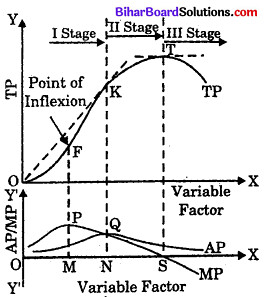In above fig. Law of Variable Proportion has been represented. Other factors being fixed, change in quantity of one factor results in three different stages of production.

First Stage : Stage of Increasing Returns : This stage has been shown till ON quantity of variable factor. At the end of this stage, MP and AP become equal to each other (see point Q). In this stage, AP increases continuously and MP is positive and greater than AP as a result of which TP increases at the increasing rate. Reasons for increasing returns to a factor is that with less amount of variable factor in the beginning, optimum utilisation of fixed factors does not become possible.

As more and more units of variable factor are employed, intensive use of fixed factors becomes possible and production increases. In this way, additional units of variable factor increases efficiency of fixed factors. Fixed factors being indivisible cannot be used in lesser amount from technical point of view. So, they cannot be used with less amount of variable factor. Hence, more units of variable factor are used and it increases production. This is called increasing retunrs. In this stage, TP curve is shown point O to point K. OK can be divided into two parts or the first stage can be divided into two parts :

(a) Point O to Point F : TP is increasing with increasing rate because MP is continuously increasing till point P. Form point O to point F, TP is convex to X-axis.

(b) Point F to Point K : Total production increases but at a decresing rate because MP decreases but is positive (see point P to point Q). TP curve between point F to point K is concave to X-axis.

Point F on Total Product curve at which MP is maximum is called ‘Point of Inflexion’. After this point, total product increases at a decreasing rate.

Second Stage : Stage of Decreasing Returns : This stage has been shown on TP curve between point K and point T. In this stage, although TP increase but at a decreasing rate because both MP and AP i.e., marginal product and average product decreases. This stage, ends at the point, where MP is zero (Point S).

When MP is zero, then TP is maximum (see point T). This stage explains that if OS uits of variable factors are used, TP will be maximum. Reasons for decreasing returns an this stage are that fixed factors of production cannot be increased in short-run. When ON units of variable factors are.used with fixed factors, then MP decreases till zero level due to over utilisation of fixed fators and as a result of which internal diseconomies occur. In other words, extensive use of variable factors with fixed factors results in decline or decrease in MP.

Third Stage : Stage of Negative Returns : In this stage, Total Production (TP) starts decreasing after point T because at point T, marginal productivity of variable factors is zero. If after point S, an additional unit or marginal unit of variable factor is used, then marginal productivity of that additional unit becames negative and as a result TP starts declining. Therefore, this stage is called ‘Stage of Negative Returns’. In this stage, variable factors exceed fixed factors. This disbalanced or unequal ratio between the two, makes marginal productivity or variable factors negative.Question 29.
Discuss the short-run cost curve with the help of diagram.
Conceptually, in the short run, the quality of at least one input is fixed and the quantities of the other inputs can be varied. In tb. short-run period, factors, such as land and machi.iury, remain the same.

On the other hand factor such as labour and capital, vary with time. In the short run, the expansion is done by living more labour and in creasing capital. Following are the cost concepts that are taken into consideration in the short-run.

I. Total Fixed cost (T.F.C) : Refer to the cost that remain fixed in the short period. These costs do not change with the change in the level of output. Therefore, the slope of TFC curve is horizontal straight line.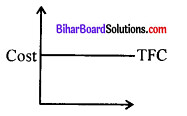II. Total variable cost (T.V.C) : Refers to the cost that change with the change in the level of production. If the output is zero, then variable cost is also zereo.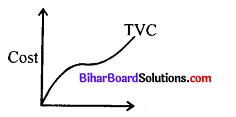III. Total Cost (T.C.) : Involves the sum of TFC and TVC ss It can be calculated as follow total cost = TFC + TVC TC also changes with the changes in the level of output-on there is a change in TVC.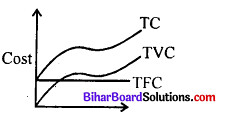IV. Average Fixed Cost (AFC) : Refers to the per unit fixed costs of production. In other words, AFC inplies fixed cost of production divided by the quantity of output produced.
AFC = TFC/Output
TFC is constant on production increase, thus AFC falls.V. Average Variable Cost (AVC) : Refer to the per unit variable cost of production. It implies organization’s variable costs divided by the quanity of output produced.
It is calculated as AVC = TVC/Outputty6VI. Average Cost (AC) : Refers to the total costs- of production per unit of output.
AC is calculated as :
AC = TC/Output
AC = TC/Output
AC is equal to the sum of total of AFC and AVC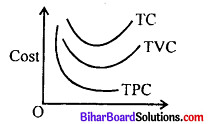VII. Marginal Cost (MC): Refers to the addition to the total cost for producing on additional unit of product.
Marginal cost is calculated as :
MC = TCN = TCn-1
n = Number of units produced
It is calculated as :
MC = ATC/A Output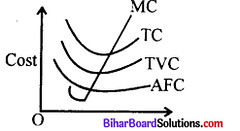Question 30.
What is Perfect Competition ? Explain the characteristics of Perfect Competition.
Perfect competition is that market situation in which a large number of buyers and sellers and found for homogeneous product single buyer or the seller are not capable of affecting the prevailing price and hence in a perfectly competition market, a single market price prevails for the commodity.
Features of Perfect Competition :

1. Large number of buyers and sellers : Perfect competition market has a large number of buyers and sellers and hence any buyer or seller can not influence the market price. In other words individual buyer or seller can not influence the demand and supply conditions of the market.
Paper
2. Homogeneous product : The units sold in the market by all sellers are homogeneous (or identical) in nature.
3. Free entry and exit of firms : In perfet competition any new firm may join the industry or any old firm may quit the industry. Hence there is no restrication on free entry or exit of firms into/form the industry.
4. Perfect knowledge of the market: In perfect competition every buyer has the perfect knowledge of market conditions. None of the buyers will buy the commodity at higher price than the prevailing price in the market. Hence only one price prevails in the market.
5. Perfect mobility of factors : In perfect competition the factors of production are perfectly mobile. Factors can easily be mobile from one industry to other industry (or one firm to another firm) without any difficulty.
6. No transportation cost : Transportation cost remains zero in perfect competition due to which one price prevails in the market.Question 31.
How the problems of double counting in computation of natonal income can be removed ?
Meaning : Double counting means counting of the value of the same product (or ependiture) more than once. How ? According to output method, of calculating national income value of only final goods and services produced by all the production units of a country during a yera should be counted. In others words, value of intermediate goods which enter into final goods Ce.g. paper used in printing of books, raw cotton used in garments, wheat used in making breads, should not be taken into acount.

But in actual practice, while taking value of final goods, value of intermediate goods also gets included because every producer treats the commodity he sells as final irrespective of weather it is used as intermediate or final good. For instance, while taking value of final goods like cycles the value of tyres, tubes, frames, bells etc. (intermediate goods) used in manufacturing these cycles also gets included inadvertently.
In this way certain items are counted more than once resulting in over estimation of national product to the extent of the value of intermediate goods included. This is called the problem of double counting which means counting value of the same commodity more than once.

How to avoid Double Counting : Theoritically we may say that there may be two alternative ways of avoiding double counting namely
(i) final product approach
But in actual practice, double counting still occurs unintentionally- in final product approach because every producer treats the product he sells as final product though the same night have been used by the buyer as an intermediate product. Therefore, this problem is perfectly solved by value added method according to this method ‘instead of taking value of finai products, value added by eacb firm at each stage of production is included.Clearly value added by all the four firms is Rs. 3500 comprising Rs. 1000 by A + Rs 500 by B + Rs. 700 by C + Rs: 1300 by D. There is no scope of double counting in this method. Thus to avoid double counting, the value added method also known as industry of origin method is used in computation of national income. It is worth mentioning that total value added is equal to value of final product.

Question 32.
What is Domestic Product ? How Gross Domestic Product is calculated ?
Domestic product referss to the money value with in of all the final goods and services produced with in the domestic territory of a country, the following equation is used to calculate the Gross Domestic product (GDP): GDP = C +1 + G (X-M) or GDP = Private consumption + Gross isnvestment + government investment + government spending + (exports – imports).

It transforms the money value measure, nominal GDP, into an index for quantity of total output.

Question 33.
Explain main points of Keynesian theory of income and employment.
According to Keynesian employment theory in short period; total production, i.e., national income in capitalist economy depends on the level of employment because in short period other factors of production such as capital, technique, etc., remain constant. Employment level depends on effective demand. That level of aggregate demand at which it becomes equal to aggregate supply is called effective demand.

Aggregate Demand : The total demand of goods and services in an economy is termed as ‘aggregate demands’ which is expressed in terms of total expenditure made in the economy. Thus, aggregate demand in an economy is measured in terms of total expenditure on goods and services.

In other words, aggregate demand refers to total expenditure that the residents of a country are ready to incur on the purchase of goods and services at given level of income.

Aggregate Demand = Consumption Expenditure + Investment Expenditure AD = C + I
Aggregate Supply : The concept of aggregate supply is related to the total supply of goods and services made available by all producers in the economy. It means that aggregate supply depends on the production made by producers.

A necessary condition of equilibrium (income level or employment level) in an economy is
Aggregate Demand = Aggregate Supply C+I=C+S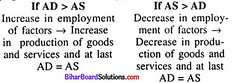Classical viewpoint explains the income equilibrium level at full employment level, i.e., aggregate demand can equal aggregate supply only at a point of full employment.

Countrary to it, according to Keynesian viewpoint aggregate demand equals aggregate supply at the point before full employment level (i.e., taken place at underfull employment level). Aggregate demand has to be increased for increasing employment in the economy.i.e. sin Keynesian viewpoint, under-full employment, equilibrium can be converted to full employment equilibrium only by increasing aggregate demand.

According to Keynes, “Under employment equilibrium is a situation in which aggregate demand (which is less than full employment aggregate demand) is equal to aggregate supply so that there is equilibrium but some resources remain unemployed.”

In this situation, factors are not fully employed and all laborers do not get employment. According to Keynes, under employment situation appears due to deficient aggregate demand but not due to deficiency of aggregate supply.
In above Fig. at ON 1 employment level, AD = AS but it is a situation of under-full employment. When aggregate demand AD increases to AD|, full employment level On is obtained.

error: Content is protected !!